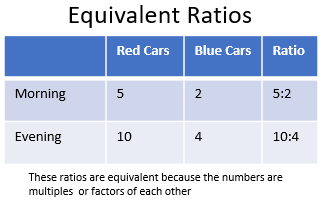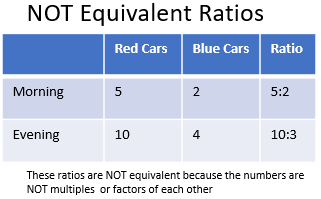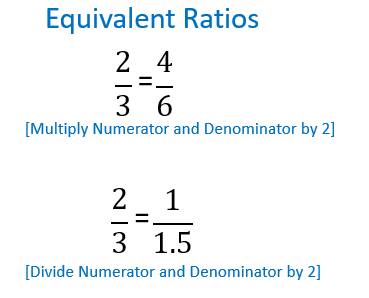# Equivalent Ratios & Examples | What are Equivalent Ratios?

Joseph Vigil, Dawn Mills
• Instructor
Joseph Vigil

Joseph has a master's degree in literature as well as alternative teaching and ESL educator certifications. He has worked with middle school, high school, and college students in writing and language arts.

• Expert Contributor
Dawn Mills

Dawn has taught chemistry and forensic courses at the college level for 9 years. She has a PhD in Chemistry and is an author of peer reviewed publications in chemistry.

Learn about equivalent ratios, including the equivalent ratios definition and examples. Learn how to find equivalent ratios and where to put these skills into use. Updated: 05/09/2021

Show

## What Are Equivalent Ratios?

A ratio is a mathematical way of comparing two quantities. The first quantity to be compared is written first, and the second quantity to be compared is written second. For example, at 8 AM, there are 5 red cars and 2 blue cars in a parking lot. In this case, the ratio of red cars to blue cars is 5 to 2. To express this in ratio form, it can be written in two different ways, 5:2 or 5/2. The ratio of blue cars to red cars is 2 to 5, which can be written 2:5 or 2/5.

At 5 PM, there are 10 red cars and 4 blue cars, so the ratio of red cars to blue cars is 10:4 or 10/4. Comparing this to the ratio in the morning, we can say that in mathematical terms the ratio 5/2 and 10/4 are equivalent ratios. This is because the values of the ratios are equal. You can derive 10/4 from 5/2 by dividing both numbers by 2. And you can derive 5/2 from 10/4 by multiplying both numbers by 2. In this case the ratios changed in proportion, as reflected in the following equivalent ratios table..### Equivalent Ratios Definition

Equivalent ratios are fractions that are derived from each other so that their values are equal. They can be considered as ratios that compare with each other such that one ratio is equal in value to the other ratio. The ratios are considered to be equivalent because the numerator and denominator are changed in proportion. Equivalent ratios are multiples or factors of each other.

Two ratios are equivalent if:

• When multiplying the numerator and denominator of one ratio by the same number you get the other ratio
• When dividing the numerator and denominator of one ratio by the same number you get the other ratio

Now, suppose in the evening there were 10 red cars and 3 blue cars. The ratios 5/2 and 10/3 are NOT equivalent because you cannot derive the second ratio by multiplying both numbers of the first ratio by the name number. In this case the ratios did NOT change in proportion.### Equivalent Ratios Examples

Let's look at some equivalent ratios examples. Consider a parking lot with 1 bicycle and 1 car and another parking lot with 3 bicycles and 3 cars. As the number of bicycles and cars triples, the number of wheels for the bicycles and the cars also triples. One bicycle has 2 wheels, 3 bicycles have 6 wheels. One car has 4 wheels, 3 cars have 12 wheels. The ratio of wheels for 1 bicycle to 1 car is 2/4. And the ratio of wheels for 3 bicycles to 3 cars is 6/12. We can say that 6/12 and 2/4 are equivalent ratios because when the number of bicycles tripled, the number of cars also tripled.

In mathematical terms, we can find the following pattern: when the numerator and denominator of the first ratio are multiplied by the same number 3, you get the second ratio. And when the numerator and denominator of the second ratio are divided by the same number 3, you get the first ratio.

The two ratios 2/4 and 6/12 are equivalent ratios because they represent the same value.An error occurred trying to load this video.

Try refreshing the page, or contact customer support.

Coming up next: Equivalent Sets: Definition & Example

### You're on a roll. Keep up the good work!

Replay
Your next lesson will play in 10 seconds
• 0:01 Ratios
• 0:58 What Are Equivalent Ratios?
• 2:27 What Are Equivalent Fractions?
• 3:17 Example
• 4:00 Lesson Summary
Save Save

Want to watch this again later?

Timeline
Autoplay
Autoplay
Speed Speed## How to Find Equivalent Ratios

To find an equivalent ratio, multiply the numerator and denominator of the ratio by the same number OR divide the numerator and denominator of the ratio by the same number.

Given a ratio 3:5, how can you find an equivalent ratio? The first step would be to write the ratio in the form of a fraction 3/5. Now you can find any number of equivalent ratios by multiplying the numerator and denominator by the same number.

• 3/5 = 6/10 (multiply the numerator and denominator by 2)
• 3/5 = 9/15 (multiply the numerator and denominator by 3)
• 3/5 = 30/50 (multiply the numerator and denominator by 10)
• 3/5 = 1.5/2.5 (divide the numerator and denominator by 2)
• 3/5 = 0.3/0.5 (divide the numerator and denominator by 10)

You can also find equivalent ratios from other equivalent ratios. In this example, we already have 3/5 = 30/50, so you can multiply the numerator and denominator of the equivalent ratio 30/50 by the same number 3 to get another equivalent ratio:

• 30*3 / 50*3 = 90/150

The following ratios are all equivalent ratios to each other.

• 3/5 = 6/10 = 9/15 = 30/50 = 0.3/0.5 = 90/150

You can continue this way any number of times to get an infinite number of equivalent ratios.

To summarize, the method used to find equivalent ratios or equivalent fractions is as follows:

1. Take the first ratio.
2. Multiply OR divide the numerator and denominator by the same number to get the second ratio.
3. Now take the first ratio OR the new equivalent ratio.
4. Multiply OR divide the numerator and denominator by the same number to get a new equivalent ratio.
5. Continue the process to find any number of equivalent ratios.

To unlock this lesson you must be a Study.com Member.

## Equivalent ratios

Equivalent ratios are fractions used to convert between units by representing equal measures. The exercises below will be used to assist you in further understanding the use of equivalent ratios and their role in conversions. Solutions are provided below

## Exercises

1. Are the fractions 3/20 and 9/60 equivalent?

2. What is the numerator of 3/65? What is the denominator?

3. If John takes 4 hours to do his homework, how many minutes did he take? What is the equivalent ratio to convert between minutes and hours?

4. Setup and solve the equivalent ratio for the following scenario. If Jamie was going on a road trip across the country and drove at a constant pace of 75 miles per hour, how many hours did it take him to travel 975 miles?

5. Setup and solve the equivalent ratio for the following scenario. If Betty makes \$10.50 an hour, how much money will she make after working 80 hours?

6. Is 2/9 equivalent to 6/20?

7. Setup and solve the equivalent ratio for the following scenario. Justin can eat ten mini cheeseburgers as one meal. If they cost \$1.25, how much does his meal cost?

## Solutions

1. Yes, the 3/20 is a reduced form of 9/60

2. The numerator is 3, the denominator is 65

3. It will take him 240 minutes, 1 hour = 60 minutes so the ratio is 1/60

4. 75 mi = 1 hr, 975 mi = 13 hours

5. 1 hr = \$10.50, 80 hrs = \$840

6. No

7. \$1.25 = 1 cheeseburger, 10 cheeseburgers = \$12.50

### Register to view this lesson

Are you a student or a teacher?